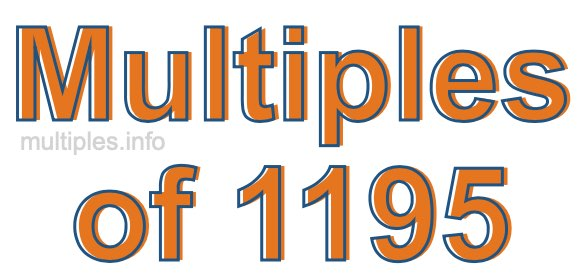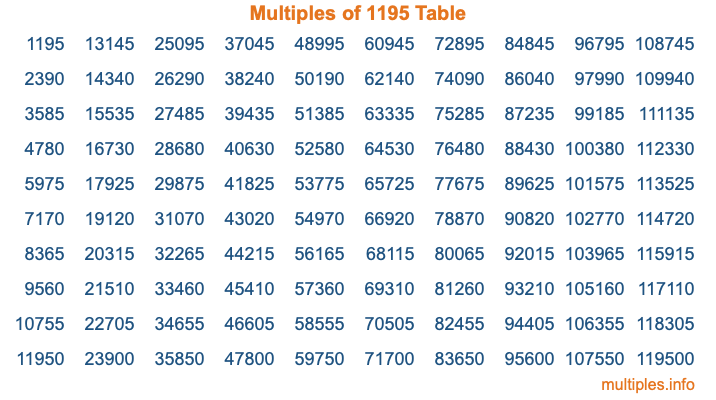Multiples of 1195Welcome to the Multiples of 1195 page. Here we will first teach you everything you will ever need to know about the multiples of 1195, and then give you a study guide summary of everything we taught you to make sure you remember it all. Use this page to look up facts and learn information about the multiples of 1195. This page will make you a multiples of one thousand one hundred ninety-five expert!

Definition of Multiples of 1195
Multiples of 1195 are all the numbers that when divided by 1195 equal an integer. Each of the multiples of 1195 are called a multiple. A multiple of 1195 is created by multiplying 1195 by an integer.

Therefore, to create a list of multiples of 1195, you start with 1 multiplied by 1195, then 2 multiplied by 1195, then 3 multiplied by 1195, and so on for as long as you want. Thus, the list of the first five multiples of 1195 is 1195, 2390, 3585, 4780, and 5975. To see a larger list of multiples of 1195, see the printable image of Multiples of 1195 further down on this page. We also have a category where you can choose any nth multiple of 1195.

Multiples of 1195 Checker
The Multiples of 1195 Checker below checks to see if any number of your choice is a multiple of 1195. In other words, it checks to see if there is any number (integer) that when multiplied by 1195 will equal your number. To do that, we divide your number by 1195. If the the quotient is an integer, then your number is a multiple of 1195.

Is  a multiple of 1195?

Least Common Multiple of 1195 and ...
A Least Common Multiple (LCM) is the lowest multiple that two or more numbers have in common. This is also called the smallest common multiple or lowest common multiple and is useful to know when you are adding our subtracting fractions. Enter one or more numbers below (1195 is already entered) to find the LCM.

Check out our LCM Calculator if you need more details about the Least Common Multiple or if you need the LCM for different numbers for adding and subtraction fractions.

nth Multiple of 1195
As we stated above, 1195 is the first multiple of 1195, 2390 is the second multiple of 1195, 3585 is the third multiple of 1195, and so on. Enter a number below to find the nth multiple of 1195.

th multiple of 1195

Multiples of 1195 vs Factors of 1195
1195 is a multiple of 1195 and a factor of 1195, but that is where the similarities end. All postive multiples of 1195 are 1195 or greater than 1195. All positive factors of 1195 are 1195 or less than 1195.

Below is the beginning list of multiples of 1195 and the factors of 1195 so you can compare:

Multiples of 1195: 1195, 2390, 3585, 4780, 5975, etc.

Factors of 1195: 1, 5, 239, 1195

As you can see, the multiples of 1195 are all the numbers that you can divide by 1195 to get a whole number. The factors of 1195, on the other hand, are all the whole numbers that you can multiply by another whole number to get 1195.

It's also interesting to note that if a number (x) is a factor of 1195, then 1195 will also be a multiple of that number (x).

Multiples of 1195 vs Divisors of 1195
The divisors of 1195 are all the integers that 1195 can be divided by evenly. Below is a list of the divisors of 1195.

Divisors of 1195: 1, 5, 239, 1195

The interesting thing to note here is that if you take any multiple of 1195 and divide it by a divisor of 1195, you will see that the quotient is an integer.

Multiples of 1195 Table
Below is an image of the first 100 multiples of 1195 in a table. The table is in chronological order, column by column. The first column has the first ten multiples of 1195, the second column has the next ten multiples of 1195, and so on.The Multiples of 1195 Table is also referred to as the 1195 Times Table or Times Table of 1195. You are welcome to print out our table for your studies.

Negative Multiples of 1195
Although not often discussed or needed in math, it is worth mentioning that you can make a list of negative multiples of 1195 by multiplying 1195 by -1, then by -2, then by -3, and so on, to get the following list of negative multiples of 1195:

-1195, -2390, -3585, -4780, -5975, etc.

Multiples of 1195 Summary
Below is a summary of important Multiples of 1195 facts that we have discussed on this page. To retain the knowledge on this page, we recommend that you read through the summary and explain to yourself or a study partner why they hold true.

There are an infinite number of multiples of 1195.

A multiple of 1195 divided by 1195 will equal a whole number.

1195 divided by a factor of 1195 equals a divisor of 1195.

The nth multiple of 1195 is n times 1195.

The largest factor of 1195 is equal to the first positive multiple of 1195.

1195 is a multiple of every factor of 1195.

1195 is a multiple of 1195.

A multiple of 1195 divided by a divisor of 1195 equals an integer.

1195 divided by a divisor of 1195 equals a factor of 1195.

Any integer times 1195 will equal a multiple of 1195.

Multiples of a Number
Here you can get the multiples of another number, all with the same attention to detail as we did for multiples of 1195 on this page.

Multiples of
Multiples of 1196
Did you find our page about multiples of one thousand one hundred ninety-five educational? Do you want more knowledge? Check out the multiples of the next number on our list!